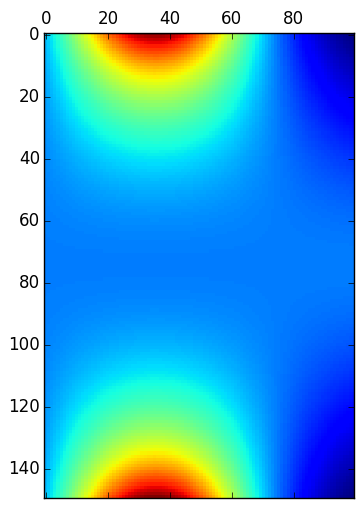# Lab 8: Function compression and the SVD¶

This lecture will explore using the SVD to compress 2D functions sampled at an evenly spaced grid. This is very much the same as image compression, but we will see that samples of smooth functions can be approximated by very small rank matrices. This gives some intuition on why pictures tend to be low rank: most pictures have large portions that are "smooth".

## 2D Functions¶

The following code samples a function on a grid in the square [-1,1]^2 and plots the corresponding pixels:

In :
using PyPlot

# We use an anonymous function here instead of a function, so that the variable f can be reused
f=(x,y)->exp(-x^2*sin(2y-1))

n=100
m=150
x=linspace(-1.,1.,m)   # sample points in x
y=linspace(-1.,1.,n)   # sample points in y

F=Float64[f(x,y) for x in x, y in y]

matshow(F);Both anonymous functions and named functions are a subtype of Function, and can be used as variables input into another function.

Exercise 1(a) Write a function fsample(f::Function,n::Integer,m::Integer) that returns a matrix of f sampled at n points in y and m points in x, both between [-1,1].

In [ ]:



Anonymous function can be used as arguments without assigning them to a variable:

F=fsample((x,y)->exp(-x^2*sin(2y-1)),100,150)


Exercise 1(b) Use fsample and matshow to plot some 2D functions for varying choices of n and m. Compare the plot of the function (x,y)->cos(100x*y) when n = m = 5?

In [ ]:



Exercise 1(c) Compare matshow to imshow on the matrix

F=fsample((x,y)->cos(100x*y),5,5)

In [ ]:



## Singular values of 2D function samples¶

We will now see experimentally that the singular values tell us something about the functions. Recall from lectures the singular value decomposition is a matrix factorization of a matrix $A \mathbb R^{n \times m}$ of the form

$$A = U \Sigma V^\top$$

where $U \in \mathbb R^{n\times n}$, $\Sigma \in \mathbb R^{n \times m}$ and $V\in \mathbb R^{m \times m}$, where $U$ and $V$ are orthogonal and $\Sigma$ is diagonal. The singular values are the diagonal entries of $\Sigma$.

Note that svdvals(A) calculates the singular values of a matrix A, without calculating the U and V components.

Exercise 2(a) Use semilogy and svdvals to plot the singular values of

F=fsample((x,y)->exp(-x^2*sin(2y-1)),100,150)

In [ ]:



Exercise 2(b) Repeat 2(a), but plotting the first 20 singular values for n=m=50 n=m=100, n=m=200 on the same figure. What do you notice? Give an explanation of why the singular values level off at approximately 1E-16.

In [ ]:



Exercise 2(c) Plot the first 50 singular values for n=m=200 of (x,y)->cos(ω*x*y) for ω equal to 1,10 and 50, on the same figure. How do the singular values change as the function becomes more oscillatory?

In [ ]:



Exercise 3(d) Plot the singular values of (x,y)->sign(x-y) for n=m=100 and n=m=200. What do you notice?

In [ ]:



Exercise 3(e) What are the singular values of eye(n)? How well can you approximate the identity matrix by a rank-r matrix?

Non-smooth functions do not have fast decaying singular values (unless the singularities are aligned to the grid). If you plot the singular values of the example image in lectures, it would look very similar.

# Thin SVD¶

In lectures, we studied the SVD, which was a decomposition of an $\mathbb R^{n \times m}$ matrix $A$

$$A = U \Sigma V^\top$$

where $U \in \mathbb R^{n\times n}$, $\Sigma \in \mathbb R^{n \times m}$ and $V\in \mathbb R^{m \times m}$, where $U$ and $V$ are orthogonal and $\Sigma$ is diagonal.

In Julia, this version of the SVD is got at using the thin=false option, where σ are the singular values (diagonal of Σ):

In :
A=rand(5,3)
U,σ,V = svd(A;thin=false)

Out:
(
5x5 Array{Float64,2}:
-0.466664   0.444423   0.438284  -0.53438    -0.327198
-0.214817   0.254689  -0.491107   0.417824   -0.687913
-0.306426  -0.563942  -0.5109    -0.564557   -0.0912669
-0.639245  -0.473705   0.387868   0.464396    0.0293991
-0.483268   0.441808  -0.394032   0.0739796   0.640721 ,

[1.8144884111170074,0.6998145559373715,0.5930904551485232],
3x3 Array{Float64,2}:
-0.645242   0.29169    0.706102
-0.46839    0.579123  -0.667253
-0.603551  -0.761271  -0.23705 )

Exercise 3(a) How do you construct Σ from σ?

In [ ]:



Exercise 3(b) Confirm numerically that the SVD satisfies the following properties:

1. U and V are orthogonal and square
2. The singular values are non-negative and listed in decreasing order
3. A - U*Σ*V' is small in norm
In [ ]:



Exercise 3(c) Repeat exercises 2(ab) for a 3 x 5 matrix.

The default SVD returned via svd is a thin SVD: it drops the vectors corresponding to the zero rows/columns of Σ so that Σ is square. (Recall the thin QR Decomposition for comparison.)

When n ≥ m, this is the factorization

$$A = \tilde U \tilde \Sigma V^\top$$

where $\tilde U \in \mathbb R^{n \times m}$, $\tilde \Sigma \in \mathbb R^{m \times m}$ and $V \in \mathbb R^{m \times m}$. When $n \leq m$, this is the factorization

$$A = U \tilde \Sigma \tilde V^\top$$

where $\tilde U \in \mathbb R^{n \times n}$, $\tilde \Sigma \in \mathbb R^{n \times n}$ and $\tilde V \in \mathbb R^{m \times n}$.

Exercise 3(d) (Advanced) Recover the full SVD from the thin SVD for both cases $n \leq m$ and $n \geq m$. (Hint: use nullspace and transposing.)

In [ ]:


In [ ]:



## Function compression¶

We now turn to using the SVD to compress functions.

Exercise 4(a) Write a function svdcompress(A::Matrix,r::Integer) that returns the best rank-r approximation to A.

In [ ]:



Exercise 4(b) Compare a matshow plot of F=fsample((x,y)->exp(-x^2*sin(2y-1)),100,100) to its rank-5 approximation.

In [ ]:


In [ ]:



Exercise 4(b) Write a function svdcompress_rank(A::Matrix,ε::Float64) that returns r so that Ar=svdcompress(A,r) satisfies norm(A-Ar) ≤ ε. (Hint: use the singular values instead of guess-and-check.)

In [ ]:



Exercise 4(c) Plot the rank needed to achieve an accuracy of 1E-4 for (x,y)->cos(ω*x*y) as a function of ω, for ω ranging from 1 to 500 and n=m=500. Can you conjecture what the rate of growth of the necessary rank is?

In [ ]: